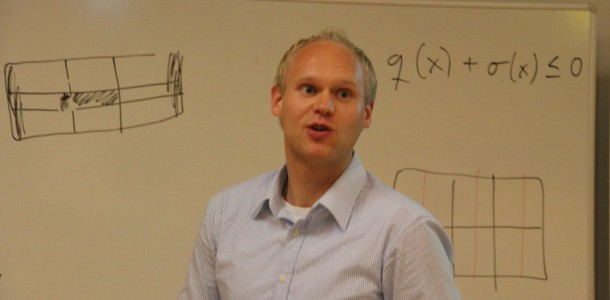###### Bayesian learning of graphical models and classifiers

April 25, 2014

During the past two decades, extensive research has shown the value and versatility of graph representations of dependence structures of multidimensional probability distributions. Such representations, generally referred to as graphical models, intertwine probability theory and graph theory. They provide a natural tool for dealing with two problems that occur throughout applied mathematics and engineering, namely uncertainty and complexity. Fundamental to the idea of a graphical model is the notion of modularity, according to which a complex system is built by combining simpler parts. Probability theory is utilized to combine such parts, ensuring that the system as a whole is consistent, and providing ways to interface models to data. The graph theoretic side of graphical models provides both an intuitively appealing interface by which humans can model interacting sets of variables as well as a data structure that lends itself naturally to the design of efficient general-purpose algorithms.

Many of the classical multivariate probabilistic systems studied in fields such as statistics, systems engineering, information theory, pattern recognition and statistical mechanics are special cases of the general graphical model formalism. Examples of such include mixture models, factor analysis, hidden Markov models, Kalman filters and Ising models. The graphical model framework provides a way to view all of these systems as instances of a common underlying formalism, see Jordan (2004). The concept of causality has also been extensively linked to graph theoretical approach by Judea Pearl and co-workers, see Pearl (2000). The most comprehensive account of the theoretical and statistical aspects of graphical models is given in the monograph by Lauritzen (1996).

A graphical model specifies a graph representation of the dependence structure of a multidimensional probability distribution, where nodes or vertices represent variables and edges or arcs (directed edges) association or influence between variables. Under viable assumptions, the graph representation enables the specification of the complete distribution in terms of conditional distributions of variables included in certain modular components of the graph. These components become identified through the explicit correspondence between conditional independence and lack of arcs or edges.

Generally, undirected graphical models, sometimes also referred to as Markov random fields, obey the following simple definition of independence. Two sets of nodes A and B are conditionally independent given a third set, C, if all paths between the nodes in A and B are separated by a node in C. By contrast, directed graphical models, also called Bayesian networks (BNs) or belief networks, have a more complicated notion of independence, which takes into account the directionality of the arcs. This can be used as a guide to construct the graph structure, and enables the notion of causality to embedded to the graph structure via regarding an arc from A to B as an indicator of A “causing” B. Bayesian learning of graph topologies has been considered by numerous authors, see, e.g. Heckerman et al. (1995) and Corander et al. (2008a). For a thorough discussion of the interplay between causality and graphical models, see Pearl (2000). However, since the specification of a real dynamic system in terms of a graphical model is even at its best a coarse description, it is preferable to assign only an operational meaning to the causalities represented by the arcs in the model.

The objectives for this research project include:

• Developing a stochastic Bayesian learning algorithm for undirected graphical models for discrete valued data. The algorithm should scale up to large sparse models, potentially harbouring tens or hundreds of thousands of nodes.
• Developing a Bayesian learning algorithm for joint clustering and graphical model learning, such that the graphical model represents dependencies between variables.
• Investigate identifiability of the generic learning problem where data are to be jointly clustered and for each cluster one aims to learn its unknown graphical model encoding dependencies between variables.

Some references

Corander, J. (2003). Labeled graphical models. Scandinavian Journal of Statistics. 30, 493-508.

Corander, J., Gyllenberg, M. and Koski, T. (2006). Bayesian model learning based on a parallel MCMC strategy. Statistics and Computing, 16, 355-362.

Corander, J., Ekdahl, M. and Koski, T. (2008). Parallell interacting MCMC for learning of topologies of graphical models. Data Mining and Knowledge Discovery, 17, 431-456.

Corander J, Marttinen, P. Bayesian identification of admixture events using multi-locus molecular markers. Molecular Ecology, 2006, 15, 2833-2843.

Ekdahl, M (2006). Approximations of Bayes classifiers for obtaining clusters. Phil.lic. thesis. University of Linköping.

Friedman, N., Geiger, D. and Goldszmidt. M. (1997). Bayesian network classifiers, Machine Learning, 29, 131-163.

Heckerman, D, Geiger, D and Chickering DM (1995) Learning Bayesian networks: The combination of knowledge and statistical data.Machine Learning 20: 197-243.

Jordan, MI (2004) Graphical models. Statistical Science 19: 140-155.

Lauritzen, SL (1996) Graphical models. Oxford: Oxford University Press.

Pearl, J (2000) Causality: Models, reasoning and inference. Cambridge, Cambridge University Press.